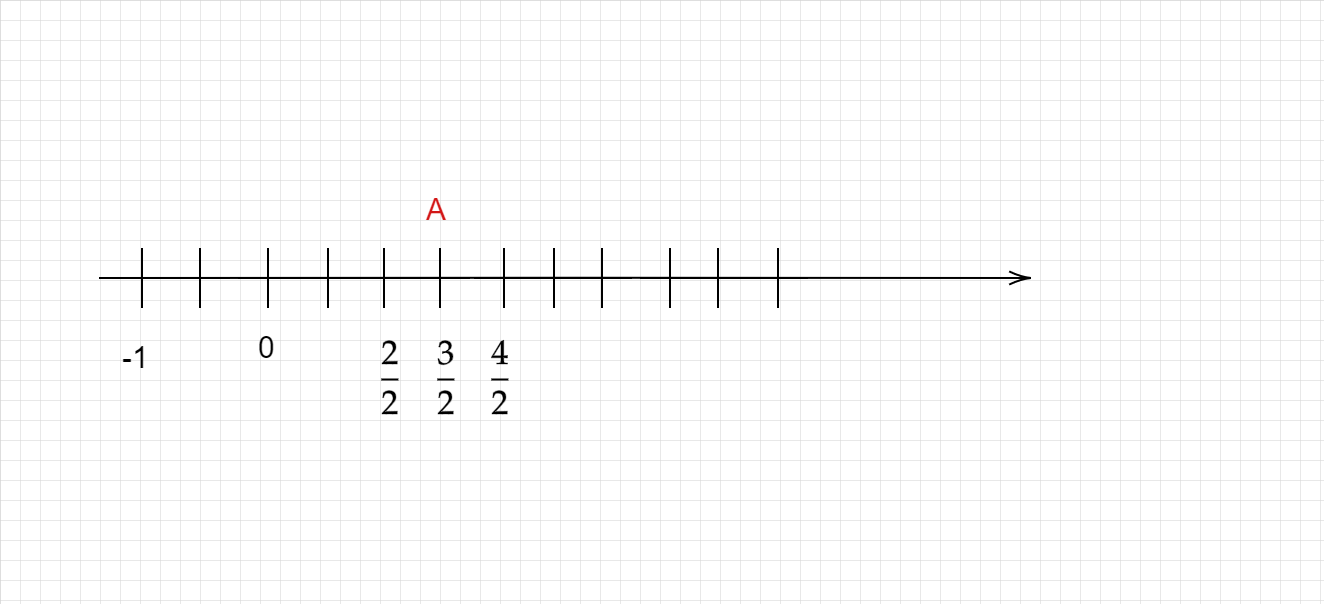# How to represent a rational number greater than 1?

Representing a rational number greater than 1 on the number line:

• To represent a rational number greater than 1 on the number line, first, convert it into a mixed fraction.
• The number will lie between the whole number part and the next whole number.
• Divide the number line between the whole number part and the next whole number into n parts, where n is the denominator of the fractional part.
Example :

Lets take $\frac{3}{2}$

Convert to mixed fraction, we get,

$\frac{3}{2} = 1\frac{1}{2}$

So, $1\frac{1}{2}$ lies between 1 and 2 in the number line.From the above figure, Point A represents the number $\frac{3}{2}$.

Updated on: 10-Oct-2022

107 Views